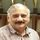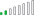cancel
Showing results for
Did you mean:Helper I

## Dax Help

| Name |  Basketball |  Tennis | Hockey |

| John   |   3              |  3          |  2          |

| Chris  |   3              |  3          |  3          |

| Pedro | 5                | 5           | 4           |

Say I only want to count rows (Name) that have scores higher than 3 and above for the all 3 columns (i.e. Basketball, Tennis and Hockey).

In this case out output would be 2 (counting Chris and Pedro), whats the best DAX equation for this?

1 ACCEPTED SOLUTIONHelper I

CheenuSing

Actually I figured it out as soon as i posted it and its similar to what you have posted.  But I ended up using the measure as per below:

All Categories 3 & Above = CALCULATE(
COUNTROWS(Table),
Table[Column1]> 2, Table[Column2]> 2, Table[Column3]>)

Thanks to everyone for thier contibution.

4 REPLIES 4Community Champion

@magtuto

Try the expression

TotRows = Calculate ( CountRows(YourTable),
Filter( ALL(YourTable),
(  YourTable[ Hockey ] >=3 &&YourTable[  Basketball ] >= 3 && YourTable[  Tennis ]
)
)
)

If this works please accept this as solution and also give KUDOS.

Cheers

CheenuSing

Did I answer your question? Mark my post as a solution and also give KUDOS !

Proud to be a Datanaut!Helper I

CheenuSing

Actually I figured it out as soon as i posted it and its similar to what you have posted.  But I ended up using the measure as per below:

All Categories 3 & Above = CALCULATE(
COUNTROWS(Table),
Table[Column1]> 2, Table[Column2]> 2, Table[Column3]>)

Thanks to everyone for thier contibution.Resolver II

Hi,

You could do as suggested and it would work. I might consider avoiding too many "IF" statements as it might cause issues with performace in large data sets especially if you go onto to use these measures in other complex measures.

A calculated column might be easiest especially if you want to deal with more than three columns.

CalColumn =

MIN ( MIN ( [Basketball], [Tennis] ), [Hockey] )

There may be a more elegant mathmatical way to get the smallest of more than three numbers but I also can't think of it right now.

Then your measure would be (assuming one row per person in this case):

CountNamesGrtr3 :=
CALCULATE (
COUNTROWS ( 'DataTable'), filter('DataTable','DataTable'[CalColumn] >= 3 )
)

You could use COUNTX and build the MIN function into the filter but I would probebly avoid that and take the additional overhead of the calculated column.

Hope this helps.

ThomasSuper User

Huh, I just ran into a similar situation last night and I ended up brute forcing it. I actually had 16 columns that I had to deal with. What I did was create measures for each column and then a calculated column that summed them all up. So, in your case you might have:

```MetricBasketBallGT3 = IF(SUM([Basketball])>3,1,0)

MetricTennisGT3 = IF(SUM([Tennis])>3,1,0)

MetricHockeyGT3 = IF(SUM([Hockey])>3,1,0)MetricAllGT3 = [MetricBasketBallGT3] + [MetricTennisGT3] + [MetricHockeyGT3]```
`CalculatedColumn = [MetricAllGT3]`

You might not need the calculated column unless you wanted to know that number for each individual. I needed it because I was computing an overall average between the 16 columns but I wanted to exclude zeros when computing that average.

It was late last night, so perhaps I just didn't have my creative thinking cap on with regard to how to solve this more elegantly...

Become an expert!: Enterprise DNA
External Tools: MSHGQM
Latest book!:
Mastering Power BI 2nd EditionDAX is easy, CALCULATE makes DAX hard...